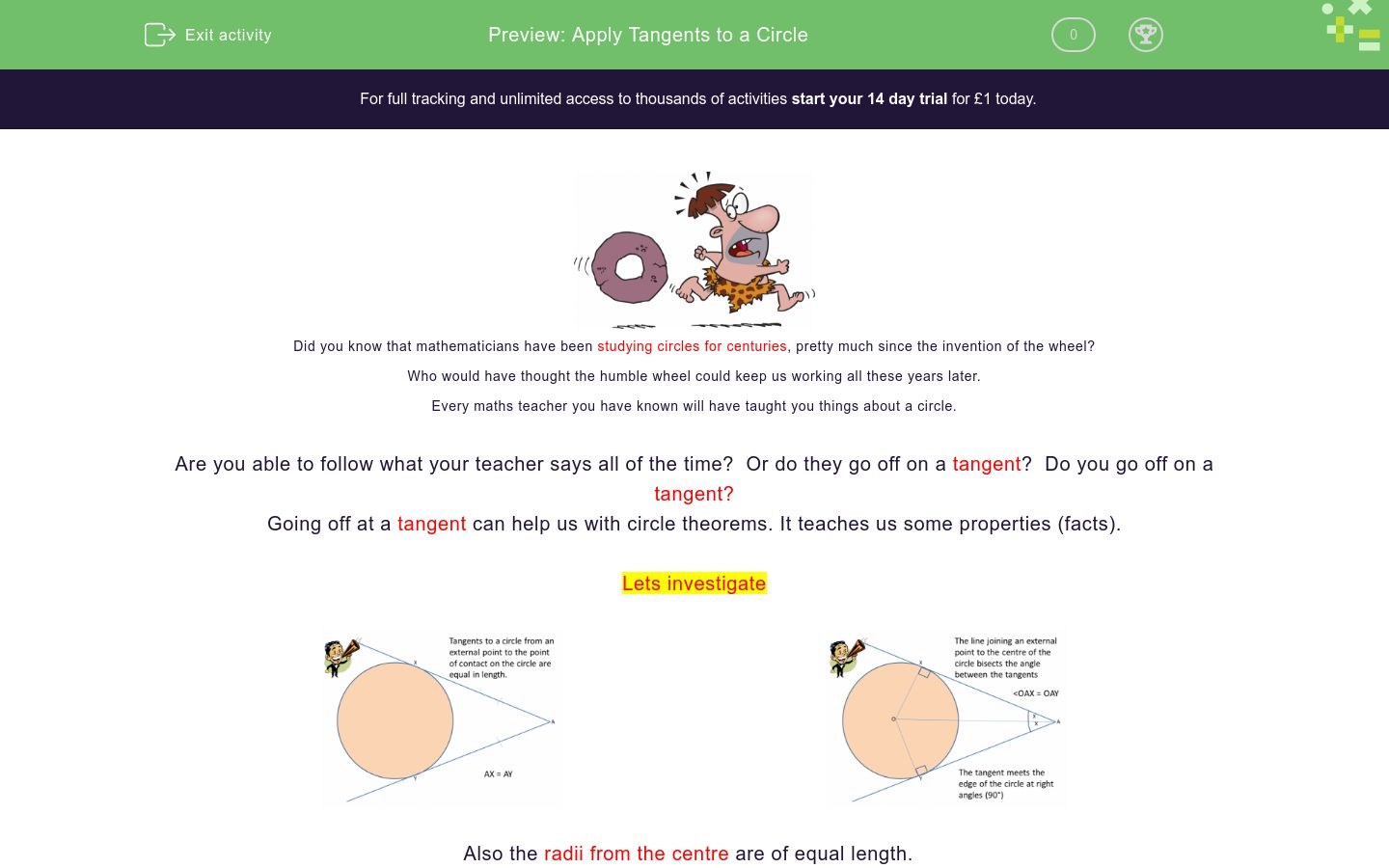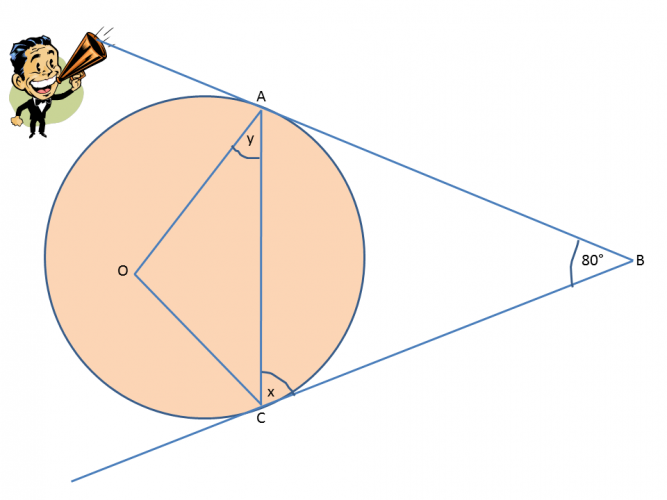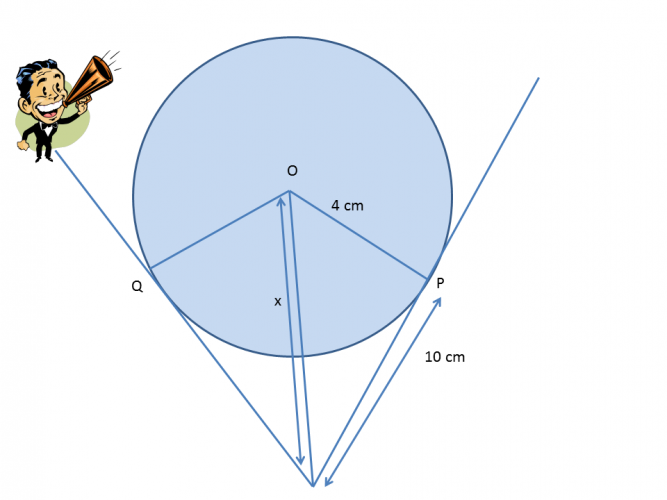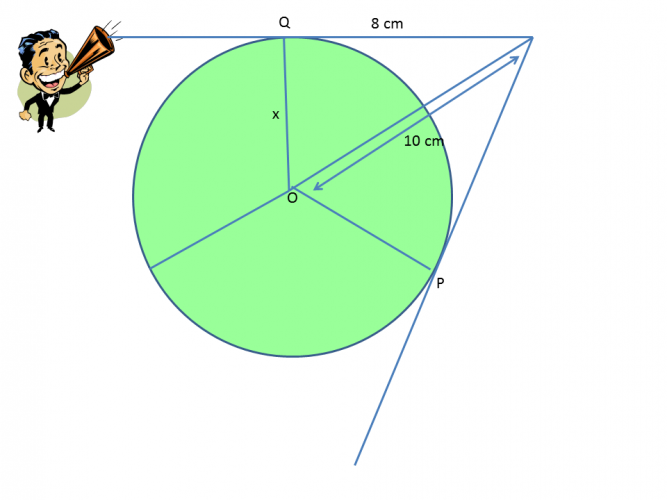# Apply Tangents to a Circle

In this worksheet, students will learn about tangents to a circle and be able to apply them.Key stage:  KS 4

GCSE Subjects:   Maths

GCSE Boards:   OCR, AQA, Eduqas, Pearson Edexcel

Curriculum topic:   Basic Geometry, Geometry and Measures

Curriculum subtopic:   Circles, Properties and Constructions

Difficulty level:### QUESTION 1 of 10Did you know that mathematicians have been studying circles for centuries, pretty much since the invention of the wheel?

Who would have thought the humble wheel could keep us working all these years later.

Every maths teacher you have known will have taught you things about a circle.

Are you able to follow what your teacher says all of the time?  Or do they go off on a tangent?  Do you go off on a tangent?

Going off at a tangent can help us with circle theorems. It teaches us some properties (facts).

Lets investigateAlso the radii from the centre are of equal length.

Can you see that a quadrilateral is formed?

These facts help us to solve problems.

Example 1Above you can see where the tangents meet the edge of the circle. This forms an isosceles triangle. (two side lengths the same and the base angles the same)

Angle x can now be worked out.

180 - 80 = 100°   100 ÷ 2 = 50°. Therefore angle x = 50°

Example 2This is one of the circle theorems that you may be given to help find a side length.

To do this you would use Pythagoras' Theorem, because right angles are formed.

To find the length OA you would apply this theorem.

12 cm² + 5 cm² = 169 cm²    √169 = 13 cmReady to chase this one down?

Note:  Circle theorems also need you to recall other properties from other basic shapes. (hint quadrilaterals, isosceles triangles)What is the value of x?

70°

90°

110°

120°90° 28° 62° 124° Angle x isWhat is the value of x?20° 30° 40° 80° 90° x is equal to 4x is equal toFind the value of x.

180°

60°

90°

45°What is the value of x?13 cm 26 cm 7 cm 8 cm The value of x isFind the value of x.Find the value of x.

12.80 cm²

6.00 cm²

18.20 cm²

8 cm²

• Question 1What is the value of x?

110°
EDDIE SAYS
Did you spot this was the same as finding the missing angle in a quadrilateral? The tangents make two right angles 90° Therefore 360 - 90 - 90 - 70 = 110° It looks more complex than it is doesn't it.
• Question 290° 28° 62° 124° Angle x is
EDDIE SAYS
The tangents form the same side lengths of the triangle. The radii are also equal length, therefore both triangles are the same. Also tangents form a quadrilateral. The missing angle at the top is therefore 28° Now we can work to find the missing angles in a quadrilateral. 360 - 28 - 28 - 90 - 90 = 124°: All we do now is to share it between two angles. x = 62°
• Question 3What is the value of x?

61
EDDIE SAYS
This is a straight forward find missing angles in an isosceles triangle. Remember the tangents make an isosceles triangle where they meet the circle. 180 - 58 = 122° 122 ÷ 2 = 61° Because the tangents do not meet a radius it is not 90°
• Question 4EDDIE SAYS
Unlike the previous question there are two radii meeting the tangent, which means it is at right angles. find the missing angle. As the triangle is isosceles x is 19° 180 - 90 - 71 = 19°
• Question 520° 30° 40° 80° 90° x is equal to 4x is equal to
EDDIE SAYS
Nothing to worry about here..honest. You know this is an isosceles triangle because of the tangents. There is no radii, so the angles are not 90°. You know base angles are the same. In total there are 9 lots of x in the triangle. You know that angles in a triangle add up to 180° 180 ÷ 9 = 20° x = 20° 4x = 80° I love a bit of algebra don't you, it simplifies everything.
• Question 6Find the value of x.

45°
EDDIE SAYS
Just to mix it up a little we have letters and numbers to work with. The radii tells us that the angles at the tangents are 90° From this we can say that 4x + 180° = 360° (angles in a quadrilateral) 4x = 180 x = 45° To check substitute in and you should have angles that add up to 360°
• Question 7What is the value of x?

10.77
EDDIE SAYS
Had you forgotten that tangents are used to help us find the lengths of sides too? The radii tells us it is a right angled triangle, so Pythagoras' Theorem can be used. 10² + 4² = 116 cm² √ 116 = 10.77 cm
• Question 813 cm 26 cm 7 cm 8 cm The value of x is
EDDIE SAYS
Are you loving the caveman for inventing the wheel or kinda wanting to throw rocks at him right now? Like anything in maths break things down into chunks. First of all find the length of the whole of the line with x in. 12 cm² + 5 cm² = 169 cm² √169 = 13 cm. You know that the radius is 5. Just subtract 5 cm from 13 cm which leaves us with 8 cm.
• Question 9Find the value of x.

3.21
EDDIE SAYS
Find the answer of the whole length of the hypotenuse. 6 cm² + 4 cm² = 52 cm² √52 = 7.21 cm 7.21 cm - 4 cm(the radius) = 3.21 cm Are you a friend of the caveman now?
• Question 10Find the value of x.

6.00 cm²
EDDIE SAYS
This was a little sneaky. This is a straightforward Pythagoras\' Theorem calculation, but a subtraction as you were finding the shorter side. 10 cm² - 8 cm² = 36 cm² √ 36 = 6 cm
---- OR ----

Sign up for a £1 trial so you can track and measure your child's progress on this activity.

### What is EdPlace?

We're your National Curriculum aligned online education content provider helping each child succeed in English, maths and science from year 1 to GCSE. With an EdPlace account you’ll be able to track and measure progress, helping each child achieve their best. We build confidence and attainment by personalising each child’s learning at a level that suits them.

Get started